## How to Calculate and Solve for Pressure Head | Aquifer Characteristics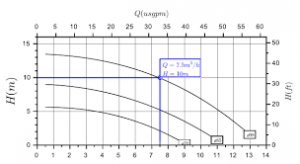The image above represents pressure head.

To compute for pressure head, three essential parameters are needed and these parameters are Pressure (P), Density (ρ) and Acceleration due to Gravity (g).

The formula for calculating pressure head:

hp = P / ρg

Where:

P = Pressure
p = Density
g = Acceleration due to Gravity

Let’s solve an example;
Find the pressure head when the pressure is 18, the density is 14 and the acceleration due to gravity is 9.

This implies that;

P = Pressure = 18
p = Density = 14
g = Acceleration due to Gravity = 9

hp = P / ρg
hp = 18 / (14)(9)
hp = 18 / 126
hp = 0.142

Therefore, the pressure head is 0.142.

Calculating the Pressure when the Pressure Head, the Density and the Acceleration due to Gravity is Given.

P = hp x pg

Where;

P = Pressure
p = Density
g = Acceleration due to Gravity

Let’s solve an example;
Find the pressure when the pressure head is 25, the density is 6 and the acceleration due to gravity is 9.

This implies that;

hp = Pressure Head = 25
p = Density = 6
g = Acceleration due to Gravity = 9

P = hp x pg
P = 25 x (6)(9)
P = 25 x 54
P = 1350

Therefore, the pressure is 1350.

## How to Calculate and Solve for Normally Occupied Positions | Ceramics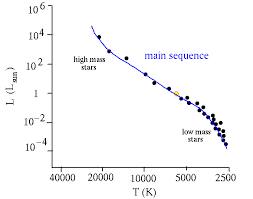The image above represents normally occupied positions.

To compute for normally occupied positions, four essential parameters are needed and these parameters are Avogadro’s number (NA), Density (ρ), Atomic weight (AK) and Atomic weights (AG).

The formula for calculating normally occupied positions:

N = NAρ / (AK + AG)

N = Normally Occupied Positions
AK, AG = Atomic Weights
ρ = Density

Let’s solve an example;
Find the normally occupied positions when the avogadro’s number is 6.022e+23, atomic weight is 12, atomic weight is 16 and the density is 10.

This implies that;

NA = Avogadro’s Number = 6.022e+23
AK, AG = Atomic Weights = 12, 16
ρ = Density = 10

N = NAρ / (AK + AG)
N = (6.022e+23)(10)/(12 + 16)
N = (6.022e+24)/(28)
N = 2.15e+23

Therefore, the normally occupied positions is 2.15e+23.

Calculating the Density when the Normally Occupied Positions, the Avogadro’s Number, the Atomic Weights is Given.

ρ = N (AK + AG) / NA

Where;

ρ = Density
N = Normally Occupied Positions
AK, AG = Atomic Weights

Let’s solve an example;
Given that normally occupied positions is 20, the avogadro’s number is 6.022e+23, atomic weights is 18, 6.

This implies that;

N = Normally Occupied Positions =20
NA = Avogadro’s Number = 6.022e+23
AK, AG = Atomic Weights = 18, 6

ρ = N (AK + AG) / NA
ρ = 20 (18 + 6) / 6.022e+23
ρ = 20 (24) / 6.022e+23
ρ = 480 / 6.022e+23
ρ = 7.970e-22

Therefore, the density is 7.970e-22.

## How to Calculate and Solve for MBAL Gas Solubility | The Calculator Encyclopedia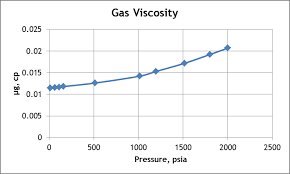The image above represents the MBAL gas solubility.

To compute for the MBAL gas solubility, four essential parameters are needed and these parameters are Specific Gravity of Solution Gas (γg), Specific Gravity of Stock Tank Oil (γo), Oil FVF (Bo) and Oil Density (ρo).

The formula for calculating MBAL gas solubility:

Rs = Boρo – 62.4γo / 0.0136γg

Where:

Rs = MBAL Gas Solubility
γg = Specific Gravity of Solution Gas
γo = Specific Gravity of Stock Tank Oil
Bo = Oil FVF
ρo = Oil Density

Let’s solve an example;
Given that specific gravity of solution gas is 9, specific gravity of stock tank oil is 13, oil FVF is 19 and oil density is 27. Find the MBAL gas solubility?

This implies that;

γg = Specific Gravity of Solution Gas = 9
γo = Specific Gravity of Stock Tank Oil = 13
Bo = Oil FVF = 19
ρo = Oil Density = 27

Rs = Boρo – 62.4γo / 0.0136γg
Rs = 19 x 27 – 62.4 x 13 / 0.0136 x 9
Rs = 513 – 811.19 / 0.1224
Rs = -298.19 / 0.1224
Rs = -2436.27

Therefore, the MBAL gas solubility is -2436.27.

## How to Calculate and Solve for Fluid Potential, Pressure, Datum Level and Density | The Calculator Encyclopedia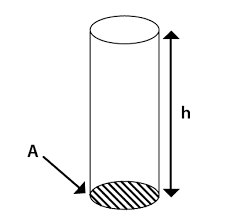The image above represents the fluid potential.

To compute for the fluid potential, three essential parameters are needed and these parameters are pressure (P), Datum Levels (ΔZ) and Density (ρ).

The formula for calculating fluid potential:

φ = P – [ρ / 144]ΔZ

Where;
φ = Fluid Potential
P = Pressure
ΔZ = Datum Levels
ρ = Density

Let’s solve an example;
Find the fluid potential when the pressure is 24 with a datum level of 18 and the density of 30.

This implies that;
P = Pressure = 24
ΔZ = Datum Levels = 18
ρ = Density = 30

φ = P – [ρ / 144]ΔZ
φ = 24 – [30/144] 18
φ = 24 – [0.2083] 18
φ = 24 – 3.75
φ = 20.25

Therefore, the fluid potential is 20.25.

Calculating the Pressure(P) when the fluid potential, Datum levels and Density is Given.

P = φ – [ρ / 144]ΔZ

Where;
P = Pressure
φ = Fluid Potential
ΔZ = Datum Levels
ρ = Density

Lets solve an example;
Find the pressure with a fluid potential of 40 and a datum levels of 18 with density of 24.

This implies that;
φ = Fluid Potential = 40
ΔZ = Datum Levels = 18
ρ = Density = 24

P = φ – [ρ / 144]ΔZ
P = 40 – [24 / 144]18
P = 40 – [0.167]18
P = 40 – 3
P = 37

Therefore, the pressure is 37.

## How to Calculate and Solve for Mass, Volume and Density | The Calculator Encyclopedia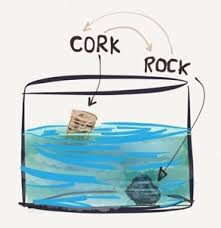The image above represents density.

To compute for the density, two essential parameters are needed and these parameters are mass (m) and volume (v).

The formula for calculating density:

Density = mass / volume

Let’s solve an example;
Given that the volume is 20 m³ with a mass of 240 kg. Find the density?

This implies that;
Volume = 20
Mass = 240

Density = mass / volume
Density = 240 / 20
Density = 12

Therefore, the density is 12 Kg/m³.

Calculating the Mass when the Density and Volume is Given.

Mass = Volume x Density

Let’s solve an example;
With a density of 90 kg/m³ and a volume of 15 m³, Find the mass?

This implies that;
Density = 90
Volume = 15

Mass = Volume x Density
Mass = 15 x 90
Mass = 1350

Therefore, the mass is 1350 kg.